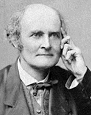Go to content
If a 2x2 matrix M has eigenvalues \lambda_1 and <\lambda_2 and corresponding eigenvectors \left(\begin{array} & s_1 \\ t_1 \end{array} \right) and \left(\begin{array} & s_2 \\ t_2 \end{array} \right),
then M can be expressed in the form S\Lambda S^{-1}, where S = \left(\begin{array} & s_1 & s_2 \\ t_1 & t_2 \end{array} \right) and \Lambda = \left(\begin{array}{rr} \lambda_1 & 0 \\ 0 & \lambda_2 \end{array} \right) .
This is called reducing M to diagonal form.

## Summary/BackgroundArthur Cayley (1821 � 1895) was a British mathematician. He helped found the modern British school of pure mathematics. As a child, Cayley enjoyed solving complex maths problems for amusement. He entered Trinity College, Cambridge, where he excelled in Greek, French, German, and Italian, as well as mathematics. He worked as a lawyer for 14 years. He published over 900 papers and notes covering nearly every aspect of modern mathematics. The most important of his work is in developing the algebra of matrices, work in non-euclidean geometry and n-dimensional geometry.
He was consequently able to prove the Cayley-Hamilton theorem -- that every square matrix is a root of its own characteristic polynomial. He was the first to define the concept of a group in the modern way -- as a set with a binary operation satisfying certain laws. Formerly, when mathematicians spoke of "groups", they had meant permutation groups.
In 1925, it was discovered that matrices and complex numbers were a necessary tool in describing the behaviour of atomic systems.You can get a better display of the maths by downloading special TeX fonts from jsMath. In the meantime, we will do the best we can with the fonts you have, but it may not be pretty and some equations may not be rendered correctly.

## Glossary

### cayley-hamilton theorem

Every square matrix satisfies its own characteristic equation.

### group

a set of elements together with a binary operation which is closed and associative, for which an identity exists in the set, and for which every element has an inverse in the set.

### matrix

a rectangular or square grid of numbers.

### permutation

a selection of r objects from a set of n unlike objects where the order of selection is important

### polynomial

an expression which has whole-numbered powers of the variable, x, multiplied by coefficients, and added together.
Examples: y = 2x, y = 1+x², y = 3-2x+7x³

### union

The union of two sets A and B is the set containing all the elements of A and B.

### work

Equal to F x s, where F is the force in Newtons and s is the distance travelled and is measured in Joules.

Full Glossary List

## This question appears in the following syllabi:

SyllabusModuleSectionTopicExam Year
AQA A-Level (UK - Pre-2017)FP4Matrix algebraCayley-Hamilton theorem-
AQA A2 Further Maths 2017Pure MathsFurther MatricesCayley-Hamilton Theorem - Extra-
AQA AS/A2 Further Maths 2017Pure MathsFurther MatricesCayley-Hamilton Theorem - Extra-
CCEA A-Level (NI)FP1Matrix algebraCayley-Hamilton theorem-
Edexcel A-Level (UK - Pre-2017)FP3Matrix algebraCayley-Hamilton theorem-
Edexcel AS Further Maths 2017Further Pure 2Matrix AlgebraCayley-Hamilton Theorem-
Edexcel AS/A2 Further Maths 2017Further Pure 2Matrix AlgebraCayley-Hamilton Theorem-
Methods (UK)M5Matrix algebraCayley-Hamilton theorem-
OCR A-Level (UK - Pre-2017)FP1Matrix algebraCayley-Hamilton theorem-
OCR A2 Further Maths 2017Pure CoreFurther MatricesCayley-Hamilton Theorem - Extra-
OCR MEI A2 Further Maths 2017Extra PureMatricesCayley-Hamilton Theorem-
OCR-MEI A-Level (UK - Pre-2017)FP2Matrix algebraCayley-Hamilton theorem-
Universal (all site questions)MMatrix algebraCayley-Hamilton theorem-
WJEC A-Level (Wales)FP1Matrix algebraCayley-Hamilton theorem-